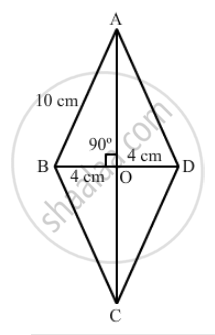Advertisement Remove all ads

# Abcd is a Rhombus Whose Diagonals Intersect at O. If Ab = 10 Cm, Diagonal Bd = 16 Cm, Find the Length of Diagonal Ac. - Mathematics

ABCD is a rhombus whose diagonals intersect at O. If  AB = 10 cm, diagonal BD = 16 cm, find the length of diagonal AC.

Advertisement Remove all ads

#### Solution$\text{ We know that the diagonals of a rhombus bisect each other at right angles }.$
$\therefore BO = \frac{1}{2}BD = (\frac{1}{2} \times 16) cm$
$= 8cm$
$AB = 10 \text{ cm and }\angle AOB = 90°$
$\text{ From right } ∆ OAB:$
${AB}^2 = {AO}^2 + {BO}^2$
$\Rightarrow {AO}^2 = ( {AB}^2 -{BO}^2 )$
$\Rightarrow {AO}^2 = (10 )^2 - (8 )^2 {cm}^2$
$\Rightarrow {AO}^2 = (100 - 64) {cm}^2 = 36 {cm}^2$
$\Rightarrow AO = \sqrt{36} cm = 6cm$
$\therefore AC = 2 \times AO = (2 \times 6) cm = 12 cm$

Is there an error in this question or solution?
Advertisement Remove all ads

#### APPEARS IN

RD Sharma Class 8 Maths
Chapter 17 Understanding Shapes-III (Special Types of Quadrilaterals)
Exercise 17.2 | Q 13 | Page 17
Advertisement Remove all ads

#### Video TutorialsVIEW ALL 

Advertisement Remove all ads
Share
Notifications

View all notifications

Forgot password?
Course